# Class 8 Maths NCERT Solutions for Chapter – 15 Introduction to Graphs Ex – 15.1

## Introduction to Graphs

Question 1.
The following graph shows the temperature of a patient in a hospital, recorded every hour.
(a)
What was the patient’s temperature at 1 p.m.?
(b) When was the patient’s temperature 38.5°C?

(c)
The patient’s temperature was the same two times during the period given. What were these two times?
(d) What was the temperature at 1.30 p.m.? How did you arrive at your answer?
(e) During which periods did the patient’s temperature showed an upward trend.

Solution:
In the graph, we find that the time (in hours) are represented on the x-axis and the temperature (in °C) are represented on the y-axis. The temperature at any time can be read from the graph exactly in the same way as we read the coordinates of a point. From the graph, we observe that :
(a) The temperature of the patient at 1 p.m. was 36.5°C.
(b) The temperature of the patient was 38.5°C at 12 noon.
(c) The temperature of the patient was same at 1 p.m. and 2 p.m.
(d) The temperature of the patient at 1.30 p.m. was 36.5°C. The point between 1 p.m. and 2 p.m. on the x-axis is equidistant from the two points showing 1 p.m. and 2 p.m., so it will represent 1.30 p.m. Similarly, the point on the y-axis, between 36°C and 37°C will represent 36.5°C.
(e) During the periods 9 a.m. to 10 a.m., 10 a.m. to 11 a.m. and 2 p.m. to.3 p.m. the patient’s temperature showed an upward trend.

Question 2.
The following line graph shows the yearly sales figures for a manufacturing company.

(a) What were the sales in
(i) 2002
(ii) 2006?

(b) What were the sales in
(i) 2003
(ii) 2005?

(c) Compute the difference between the sales in 2002 and 2006.

(d)
In which year was there the greatest difference between the sales as compared to its previous year?

Solution:
In the graph, we find that the years are represented on the x-axis and the sales (in ? crores) on the y-axis. The sales at any time (year) can be read from the graph exactly in the same way as we read the coordinates of a point. From the graph, we observe that :
(a)
(i) The sales in the year 2002 is ₹ 4 crore.
(ii) The sales in the year 2006 is ₹ 8 crore.

(b)
(i) The sales in the year 2003 is ₹ 7 crore.
(ii) The sales in the year 2005 is ₹ 10 crore.

(c) The difference between the sales in 2002 and 2006 = ₹ 8 crore – ₹4 crore = ₹ 4 crore.

(d) The year in which there was the greatest difference between the sales as compared to its previous year is the year 2005.

Question 3.
For an experiment in Botany, two different plants, plant A and plant B were grown under similar laboratory conditions. Their heights were measured at the end of each week for 3 weeks. The results are shown by the following graph :

(a)
How high was Plant A after (i) 2 weeks (ii) 3 weeks?
(b) How high was Plant B after (i) 2 weeks (ii) 3 weeks?
(c) How much did Plant A grow during the 3rd week?
(d) How much did Plant B grow from the end of the 2nd week to the end of the 3rd week?
(e) During which week did Plant A grow most?
(f) During which week did Plant B grow least? *
(g) Were the two plants of the same height during any week shown here? Specify.

Solution:
In the graph, we find that the weeks are represented on the x-axis and the heights (in cm) of two plants A and B on the y-axis. The heights on any week of plants A and B can be read from the graph exactly in the same way as we read the coordinates of a point. From the graph, we observe that :
(a) The height of the plant A after
(i) 2 weeks was 7 cm
(ii) 3 weeks was 9 cm.
(b) The height of the plant B after
(i) 2 weeks was 7 cm
(ii) 3 weeks was 10 cm.
(c) The plant A grows 2 cm during the 3rd week.
(d) The plant B grows 3 cm from the end of the 2nd week to the end of the 3rd week.
(e) The plant A grows most during second week.
(f) The plant B grows most during first week.
(g) At the end of the 2nd week the heights of the two plants were the same.

Question 4.
The following graph shows the temperature forecast and the actual temperature for each day of a week.
(a)
On which days was the forecast temperature the same as the actual temperature?
(b) What was the maximum forecast temperature during the week?
(c) What was the minimum actual temperature during the week?
(d) On which day did the actual temperature differ the most from the forecast temperature?

Solution:

In the graph, we find that the days are represented on the x-axis and the temperature forecast/actual (°C) on the y-axis. The temperature on any day can be read from the graph exactly in the same way as we read the coordinates of a point. From the graph, we observe that :
(a) The days on which the forecast temperature was the same as the actual temperature are Tuesday, Friday and Sunday.
(b) The maximum forecast temperature during the week was 35°C.
(c) The minimum actual temperature during the week was 15°C,
(d) The actual temperature differed the most from the forecast temperature on Thursday.

Question 5.
Use the tables below to draw linear graphs.
(a)
The number of days a bill side city received snow in different years.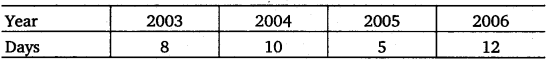(b) Population (in thousands) of men and women in a village in different years.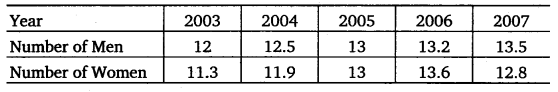Solution:
(a) In order to draw the required graph, we represent years on the x-axis and the days on the y-axis. We first plot the ordered pairs (2003, 8), (2004,10), (2005, 5) and (2006, 12) as points and then join them byline segments as shown below :

(b)
In order to draw the required graph, we represent years on the x-axis and the population (in thousands) on the y-axis. The dotted line shows the population (in thousands) of men and the solid line shows the population (in thousands) of women.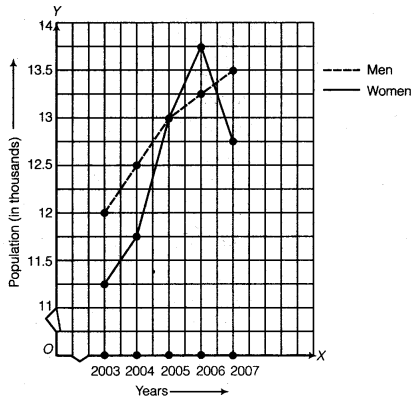We first plot the ordered pairs (2003; 12), (2004, 12.5), (2005, 13), (2006, 13.2) and (2007, 13.5) and then join them by the dotted line as shown to get the graph representing the number of men. Further, we plot (2003, 11.3), (2004, 11.9), (2005, 13), (2006, 13.6) and (2007, 12.8) and then join them by line segments as shown to get the graph representing the graph of number of women.
Thus, the required graph is obtained.

Question 6.
A courier-person cycles from a town to a neighbouring suburban area to deliver a parcel to a merchant. His distance from the town at different times is shown by the following graph :
(a)
What is the scale taken for the time axis?
(b) How much time did the person take for the travel?
(c) How far is the place of the merchant from the town?
(d) Did the person stop on his way? Explain.
(e) During which period did he ride fastest?

Solution:
In the graph, we find that the time (in hours) is represented on the x-axis and the distance (in km) is represented on the y-axis. The distance at any time can be read from the graph exactly in the same way as we read the coordinates of a point. From the graph, we observe that :
(a) The scale taken for the time axis is : 4 units = 1 hour.
(b) The person took 3$\frac { 1 }{ 2 }$ hours for the travel.
(c) The merchant’s place from the town is 22 km.
(d) Yes; this is indicated by the horizontal part of the graph (10 a.m. -10.30 a.m.)
(e) Between 8 a.m. and 9 a.m. he ride faster.

Question 7.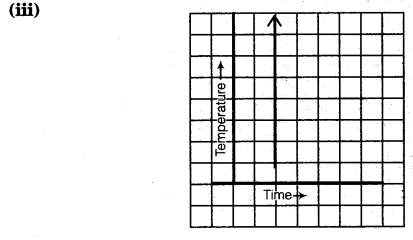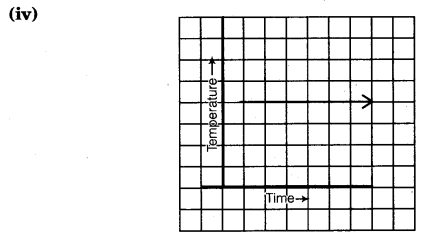Solution:
(i) This represents a time-temperature graph because it represents a smooth rise in temperature and is represented by a line graph.
(ii) This represents a time-temperature graph because it represent a smooth fall in temperature and is represented by a line graph.
(iii) This does not represent a time-temperature because it shows different temperatures at the same time.
(iv) This represents a time-temperature graph because it shows a constant temperature at different times and is a line graph.